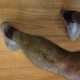# Angle Problem #1If θ = δ – 10o

find the angles.

(assume red triangles are similar, larger triangle sides are tangents to the circle and yellow shape is a rectangle)

Advertisements

## 4 thoughts on “Angle Problem #1”

1.workedgechaos says:

Think I’ve sussed this one. The key bit is the circle.

•solvemymaths says:

I’m in the middle of writing all my solutions into one nice book. I think the answer is 55 and 65 degrees. Can’t remember where the answer is at this moment though!

2.Maths Tyn says:

I got the same: 55 and 65 degrees.
Accessible when you have circle theorems.

•solvemymaths says:

correct! Well done 🙂